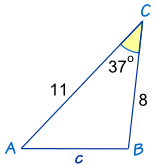# Week 17- Cos Law

This week, I have learned how to find the missing angle or missing length by using cos law.

There are 2 ways to use the cos law.

1. To find the missing angle
2. To find the missing lenght

To find the missing angle, we use : cosC=$a^2+b^2-c^2$$\div 2ab$

To find the missing length, we use :$c^2=a^2+b^2-2ab$ cos C

Let’s try an exampleWe know that angle C is 37, and we are looking for the length C.

Lets write down every thing in the formula.

Length:$c^2=a^2+b^2-2ab$ cos C$c^2=11^2+8^2-2(11)(8)$ cos 37

C= 3.9 cm

Lets try another example.Angle:

cosC=$a^2+b^2-c^2$$\div 2ab$

cos X=$6^2+5^2-8^2\div$2(6)(5)

X=$6^2+5^2-8^2\div$2(6)(5) * cos^-1

X=93